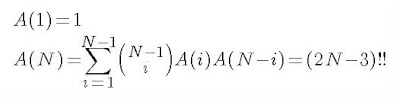## Monday, August 09, 2010

So, here's a follow-up to my previous post: Combinatorial Madness.
A follow-up to this follow-up post can be found here: Combinatorial Madness - Final Post.

After a fair bit more work at this and thinking about it (with a good deal of help from a few colleagues), here's where we have arrived. We basically have a good explanation of how we can formulate a recursive solution to the problem. And, it now comes to the point where we have to verify the following conjecture. We believe it holds true, but haven't yet proven it nor tried to prove it.

Assuming it's true, it is a pretty cool double factorial identity.

Here it is.Note that A(k) is the solution for the potion problem where N=k.

So, any takers? We haven't tried to prove the above, but basically, if you can prove that then that basically proves that the solution to the original potions problem follows the double factorial.

#### 1 comment: